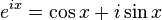# Eulers FormulaIs this true:

$$e^{i(a+bx)}=cos(a+bx)+i sin(a+bx)$$ ?

Last edited by a moderator:

chroot
Staff Emeritus
Gold Member
Yes -- that's the beauty of function notation. You can replace 'x' with any expression you like.

- Warren

Thanks

I know Daves question is answered, but instead of making a new thread I just ask another one here as it is somewhat related. Unfortunately I don't have a mathtype program so you'll have to imagine.
So if you have the e^xi expression and set x = pi, this will equal -1. Adding 2Pi equals 1. So far so good? But here comes what I don't understand. 1 can also be written e^0, so using logarithms we say: e^i2Pi = e^0 -> i2Pi = 0, but this can't be true as i2Pi = 6,28i. I got one possible solution from my math teacher but it did not quite tell me how it could equal 0. Anyone care to give an explenation a shot?

My best guess would be that the exponential function is not a bijection over the complex numbers, while it is so over the real numbers. That is, for real numbers,

e^x = e^y <=> x = y is true.

For complex numbers, it must be the case that

e^x = e^y <=> x = y is false.

This shouldn't be so surprising. For the real numbers, you can't say things like...

x^2 = y^2 <=> x = y
sin(x) = sin(y) <=> x = y
...

I suppose it's just an accident that you can't do that for the exponential function over complex numbers.

dx
Homework Helper
Gold Member
My best guess would be that the exponential function is not a bijection over the complex numbers, while it is so over the real numbers. That is, for real numbers,

e^x = e^y <=> x = y is true.

You mean injection.

Well, injection may be true too, but it's definitely not a bijection either.

I tend to talk in terms of bijection or not bijection. I usually don't delve into things like injectivity and surjectivity. I think that the exponential is neither bijective nor injective over the complex numbers... then again, what do I know?

dx
Homework Helper
Gold Member
You said exp was a bijection over the reals which it isn't. It's an injection, but not a bijection.

The problem is not with the exponential function, but with the logarithmic function. You need to define a branch cut to define the logarithmic function. This then means that the imaginary part of the Log of a complex number (the so called argument) is a unique number in some interval of length 2 pi.

Yeah, what Count Ibis said:
The problem is not with the exponential function, but with the logarithmic function. You need to define a branch cut to define the logarithmic function. This then means that the imaginary part of the Log of a complex number (the so called argument) is a unique number in some interval of length 2 pi.
For the less mathematically versed, this is a little like taking the square root of a number and having to remember that there are actually two roots (+/-). When you take the log of a complex number, there are (potentially) multiple correct results, so you have to specify which one you're choosing.

(At least, I think that's a correct statement; someone please correct me if it's not.)

"You said exp was a bijection over the reals which it isn't. It's an injection, but not a bijection."

Oops. You're right. I guess that's the price you pay when you get too used to using the general terminology too often.

The trouble is that the complex exponential function is periodic, so saying that e^x = e^y iff x=y for complex x and y is akin to saying that cos x = cos y iff x=y.